×
Get Full Access to UW - AMATH 301 - Class Notes - Week 2
Get Full Access to UW - AMATH 301 - Class Notes - Week 2

×

UW / Applied Mathematics / AMATH 301 / What is the difference between matrix operation and component operatio

# What is the difference between matrix operation and component operatio Description

##### Description: Notes from week 2: Norms (definitions and different types) and techniques of solving linear systems (Ax=b) through Gaussian elimination, LU decomposition, Matlab "\" command.
13 Pages 72 Views 4 Unlocks
Reviews

Matrix Operation  vs. Component Operations

Example: We also discuss several other topics like What does central dogma do?

1.>> A = [ 1, 3, 3, 5, 6, 7, 8, 3, 1 ];

A ^ 2   % A² = A.A  ( matrix multiplication) If you want to learn more check out How can one calculate relative prices and what do they mean?

Ans: 32      27       25Don't forget about the age old question of What does prenatal care include?

91      72       59

31      45       38Don't forget about the age old question of What is the concept of arousal theory?
If you want to learn more check out What is the conecept of durkheim's theory?

2. A ^ 2    %  squares each component

Ans: 1      9       4Don't forget about the age old question of What is the concept of james lange's theory of emotion in psychology?

25    36      44

64     9        1

3. A./A     % divides each component by corresponding component in A

Ans:   1      1      1

1      1      1

1      1      1

4. A/A  % outputs solution to Ax = A (Output: x)

Ans:    1   0     0

0   1     0

0   0     1

Max command:

[ max_vector, location] = max (A)

max _vector = 8 6 7 - max element in each column

Location: = 3 2 2 - where in column max appears

>> [ max_vector2 , location 2] = max (A, [   ], 2) finds max in each row

>> [ max_whole mat, location 3 ] = max (A (:)) finds mox in whole matrix

→ A (:) outputs A as a vector

Norms

A generalized definition at distance → different types of norms, different types of distance

Ex. Euclidean Distance (2-norm)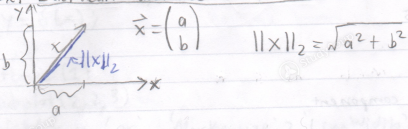1- norm (Taxi-cab/ Manhattan Distance)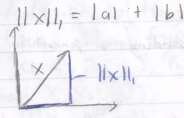∞- norm ( infinity/ max-norm)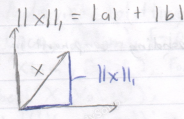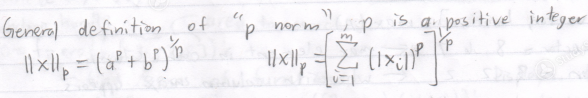Norms in MotLab

>> b = [ I, o ,-4 ]

1 >> norm (b) % Motlab default is 2-norm, norm (b) is same as norm (b,2)

Ans: 4,1231

2>> norm (b, 1)

Ans: 5

3>> norm (b, int) % in Motlab int is ∞

Ans: 4

4>> max labs (b)  % abs (b) is lbl (absolute value)

Ans: 4

-Note: norm (b, inf)= max (abs (b))

Complex Numbers

>>c = [ 3 + 4 x i, 2, 3 ,5 x i ]

1>> norm (c, int)

Ans: 5

2>>norm (c, 2)

Ans: 6.3

3>> Mox (c) % 5 i is longest element

Ans: 5 i

4>> mox (abs (c) % 15 il = 5

Ans: 5

Matrix Norms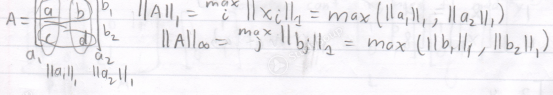Ex. >> A = [ 1, 3, 2, 2, 0, -5, 8, -2, 1 ]

1>> Norm (A , 1 )

Ans: 11

2>> Norm (A, int)

Ans: 11

Matrix 2 - norm

Given matrix A, how does it act on a unit circle. Vectors that define unit circle are rotated and scaled.

IIAII2 = length of longest semi- axis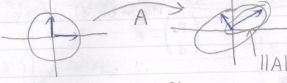>> norm (A, 2 ) % in Motlab, matrix norm is called same way as vector norm

Ans = 8.4787

Solving Linear System

Eq 1:  2x1  +   4x2  -  2x3   =2

Eq 2:  4x1  +   9x2  -  3x3 = 8

Eq 3: -2x1    -   3x2   -  7x3 = 10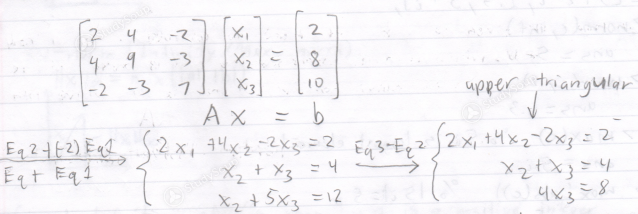Then back substitute, yet x3= 2, x2, x1 = -1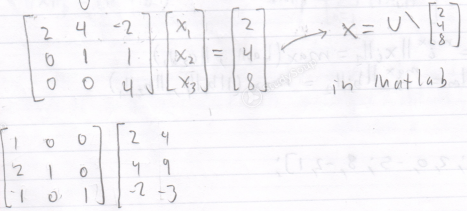In Matrix Form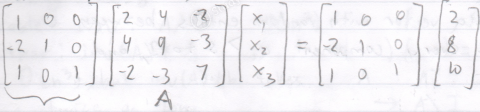Same as eq. Form takes linear combination of rows of A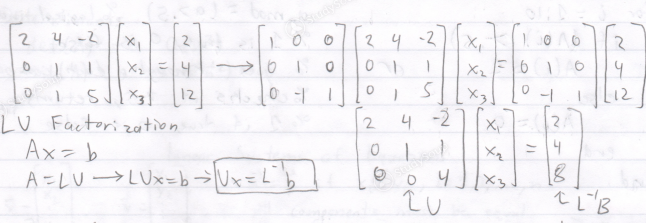Advantage in given new b, just need to compute L⁻¹, already computed L and V, which is the expensive part. This makes it faster and cheaper than Gaussian elimination, which has to do the entire process every time.

Page ExpiredIt looks like your free minutes have expired! Lucky for you we have all the content you need, just sign up here